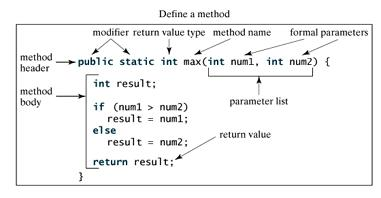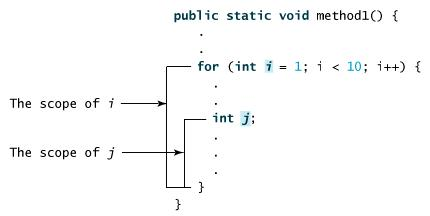# Java 方法

println()是一个方法(Method)，而System是系统类(Class)，out是标准输出对象(Object)。这句话的用法是调用系统类System中的标准输出对象out中的方法println()。

### 那么什么是方法呢？

Java方法是语句的集合，它们在一起执行一个功能。

• 方法是解决一类问题的步骤的有序组合
• 方法包含于类或对象中
• 方法在程序中被创建，在其他地方被引用

## 方法的定义

```修饰符 返回值类型 方法名 (参数类型 参数名){
...
方法体
...
return 返回值;
}
```

• 修饰符：修饰符，这是可选的，告诉编译器如何调用该方法。定义了该方法的访问类型。
• 返回值类型 ：方法可能会返回值。returnValueType是方法返回值的数据类型。有些方法执行所需的操作，但没有返回值。在这种情况下，returnValueType是关键字void
• 方法名：是方法的实际名称。方法名和参数表共同构成方法签名。
• 参数类型：参数像是一个占位符。当方法被调用时，传递值给参数。这个值被称为实参或变量。参数列表是指方法的参数类型、顺序和参数的个数。参数是可选的，方法可以不包含任何参数。
• 方法体：方法体包含具体的语句，定义该方法的功能。```public static int age(int birthday){...}
```

```static float interest(float principal, int year){...}
```

### 实例

```/** 返回两个整型变量数据的较大值 */
public static int max(int num1, int num2) {
int result;
if (num1 > num2)
result = num1;
else
result = num2;

return result;
}
```

## 方法调用

Java支持两种调用方法的方式，根据方法是否返回值来选择。

```int larger = max(30, 40);
```

```System.out.println("Welcome to Java!");
```

### 示例

```public class TestMax {
/** 主方法 */
public static void main(String[] args) {
int i = 5;
int j = 2;
int k = max(i, j);
System.out.println("The maximum between " + i +
" and " + j + " is " + k);
}

/** 返回两个整数变量较大的值 */
public static int max(int num1, int num2) {
int result;
if (num1 > num2)
result = num1;
else
result = num2;

return result;
}
}
```

```The maximum between 5 and 2 is 5
```

main方法的头部是不变的，如例子所示，带修饰符public和static,返回void类型值，方法名字是main,此外带个一个String[]类型参数。String[]表明参数是字符串数组。

## void 关键字

### 示例

```public class TestVoidMethod {

public static void main(String[] args) {
}

public static void printGrade(double score) {
if (score >= 90.0) {
System.out.println('A');
}
else if (score >= 80.0) {
System.out.println('B');
}
else if (score >= 70.0) {
System.out.println('C');
}
else if (score >= 60.0) {
System.out.println('D');
}
else {
System.out.println('F');
}
}
}
```

```C
```

## 通过值传递参数

```public static void nPrintln(String message, int n) {
for (int i = 0; i < n; i++)
System.out.println(message);
}
```

### 示例

```public class TestPassByValue {

public static void main(String[] args) {
int num1 = 1;
int num2 = 2;

System.out.println("Before swap method, num1 is " +
num1 + " and num2 is " + num2);

// 调用swap方法
swap(num1, num2);
System.out.println("After swap method, num1 is " +
num1 + " and num2 is " + num2);
}
/** 交换两个变量的方法 */
public static void swap(int n1, int n2) {
System.out.println("\tInside the swap method");
System.out.println("\t\tBefore swapping n1 is " + n1
+ " n2 is " + n2);
// 交换 n1 与 n2的值
int temp = n1;
n1 = n2;
n2 = temp;

System.out.println("\t\tAfter swapping n1 is " + n1
+ " n2 is " + n2);
}
}
```

```Before swap method, num1 is 1 and num2 is 2
Inside the swap method
Before swapping n1 is 1 n2 is 2
After swapping n1 is 2 n2 is 1
After swap method, num1 is 1 and num2 is 2
```

## 方法的重载

```public static double max(double num1, double num2) {
if (num1 > num2)
return num1;
else
return num2;
}
```

Java编译器根据方法签名判断哪个方法应该被调用。

## 变量作用域

for循环的初始化部分声明的变量，其作用范围在整个循环。## 命令行参数的使用

### 实例

```public class CommandLine {

public static void main(String args[]){
for(int i=0; i<args.length; i++){
System.out.println("args[" + i + "]: " +
args[i]);
}
}
}
```

```java CommandLine this is a command line 200 -100
```

```args: this
args: is
args: a
args: command
args: line
args: 200
args: -100
```

## 构造方法

### 实例

```// 一个简单的构造函数
class MyClass {
int x;

// 以下是构造函数
MyClass() {
x = 10;
}
}
```

```public class ConsDemo {

public static void main(String args[]) {
MyClass t1 = new MyClass();
MyClass t2 = new MyClass();
System.out.println(t1.x + " " + t2.x);
}
}
```

### 实例

```// 一个简单的构造函数
class MyClass {
int x;

// 以下是构造函数
MyClass(int i ) {
x = i;
}
}
```

```public class ConsDemo {

public static void main(String args[]) {
MyClass t1 = new MyClass( 10 );
MyClass t2 = new MyClass( 20 );
System.out.println(t1.x + " " + t2.x);
}
}
```

```10 20
```

## 可变参数

JDK 1.5 开始，Java支持传递同类型的可变参数给一个方法。

```typeName... parameterName
```

### 实例

```public class VarargsDemo {

public static void main(String args[]) {
// 调用可变参数的方法
printMax(34, 3, 3, 2, 56.5);
printMax(new double[]{1, 2, 3});
}

public static void printMax( double... numbers) {
if (numbers.length == 0) {
System.out.println("No argument passed");
return;
}

double result = numbers;

for (int i = 1; i <  numbers.length; i++)
if (numbers[i] >  result)
result = numbers[i];
System.out.println("The max value is " + result);
}
}
```

```The max value is 56.5
The max value is 3.0
```

## finalize() 方法

Java允许定义这样的方法，它在对象被垃圾收集器析构(回收)之前调用，这个方法叫做finalize( )，它用来清除回收对象。

finalize()一般格式是：

```protected void finalize()
{
// 在这里终结代码
}
```

### 实例

```public class FinalizationDemo {
public static void main(String[] args) {
Cake c1 = new Cake(1);
Cake c2 = new Cake(2);
Cake c3 = new Cake(3);

c2 = c3 = null;
System.gc(); //调用Java垃圾收集器
}
}

class Cake extends Object {
private int id;
public Cake(int id) {
this.id = id;
System.out.println("Cake Object " + id + "is created");
}

protected void finalize() throws java.lang.Throwable {
super.finalize();
System.out.println("Cake Object " + id + "is disposed");
}
}
```

```C:\1>java FinalizationDemo
Cake Object 1is created
Cake Object 2is created
Cake Object 3is created
Cake Object 3is disposed
Cake Object 2is disposed
```

(1)
100%

(0)
0%